# SPICE modeling of Magnetic Core from Datasheet

6. Let’s complete the design of the transformer Flyback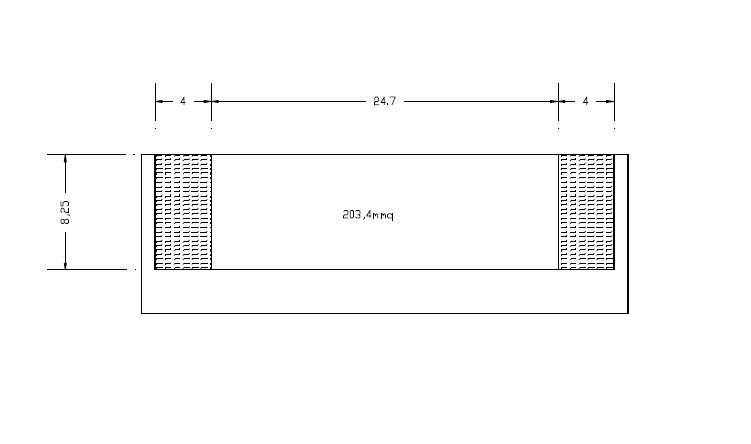Figure 9 – Detail of the support section to ETD49.

After the previous considerations let’s complete the design of the transformer Flyback converter described in Chapter 3. In Figure 9 is shown a section detail of the support for ETD49 with the relative dimensions in millimeters. Given that the rules  require a minimum lateral distance between the primary and secondary windings of 8 mm, the area available to carry out the winding will not be more that indicated in the catalog

of 269.4 mm^2, but of 203.4 mm^2. Correcting the value of the section area usable in (0.24) (0.25), we obtain: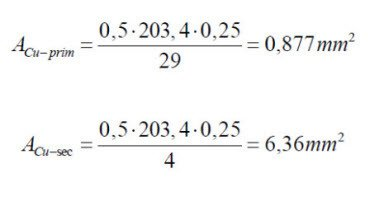Primary winding.

With the new section to the primary of 0.877 mm^2 the closer value obtained from Table 10 appears to be a wire section 0.823 mm^2 (AWG18) and a nominal diameter of 1.024 mm. The graph shown in Figure 8 shows that if we use a single conductor, the increase of the resistance for the skin effect would be greater than 5%, then excessive. Therefore, following the instructions provided in Table 9 in the appendix, it’s chosen a Litz wire with equivalent section:

18AWG – with a nominal diameter of 61mils (about 1.55 mm), resistance 7.10 Ohm/1000 feet, composed of 100 38AWG wires.

being available in the support a linear length of 24.7 mm, on a layer we could place the followinf number of turns: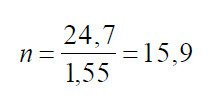therefore we’ll realize the primary with two layers, among which we’ ll interpose the secondary. To realize the most symmetrical windings possible, the 29 turns will be divided into a total number of 15 on the inner layer, and 14 on the external layer. Maintaining the value found in the manual of an average length of the coil of 86mm for the support in use, let’s estimate a total length of the primary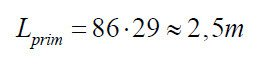Being the resistance value of 7.10 Ohm/1000 feet, or about 23.3 mOhm/m (1 feet = 30.48 cm), the resistance of the primary winding will be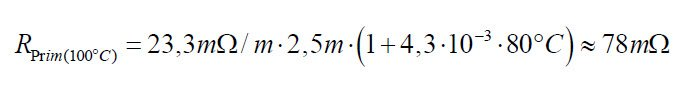neglecting losses due to proximity effect we can estimate the copper losses in the primary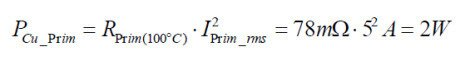Secondary winding.

With the new section to the secondary of 6.36 mm^2, the closer value obtained from Table 10 in the appendix would be the 9AWG with 6.63 mm2 cross section, but the Litz wire isn’t produced for all dimensions. In fact from Table 9 we can verify that there are only the AWG numbers even, then it’s chosen the next gauge equivalent to a section of 8.37 mm^2 ie:

8AWG – 189mils nominal diameter (about 4.8 mm), resistor 0.692 Ohm/1000 feet, composed of 1050 38AWG wires.

The secondary winding of only 4 turns, will have a linear length modest (approximately 20mm) such as to allow the realization of a single layer, for a total length of the winding ofBeing the resistance value of 0.692 Ohm/1000 feet, or about 2.27 mOhm/m (1 feet = 30.48 cm), the resistance of the secondary winding will beneglecting losses due to proximity effect we can estimate the copper losses in the secondary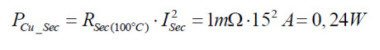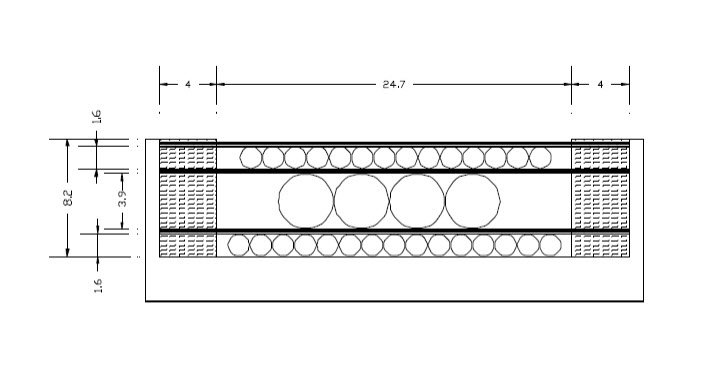Figure 10 Detail of the support section to ETD49, with the possible stratification of the windings separated by insulating layers.

In Figure 10 it is possible to observe a possible implementation of the transformer with the insulating layers interposed between the windings. Although not shown graphically, we can also interpose electrostatic shields between the windings.

Conclusions

We can see how the power dissipated by the resistance of the windings are not equivalent, about 2W lost to the primary and about 0.3 W to the secondary. We could try to balance the losses by using a larger diameter to the primary and thus reducing to the secondary. Using a 16AWG wire to the primary, the increase of diameter would be such as to not allow more to perform the winding with two layers. This, if on the one hand would be beneficial in terms of heating of the primary winding, balanced however by an increase in losses to the secondary with size wire lower,  it would increase the value of parasitic inductance for the asymmetry of the windings, so as not to justify the assumptions made.

In the case of difficulties of realization for lack of space it ‘s possible to reduce the gauge of the wire to the secondary. Using a 10AWG (5.26 mm^2) the maximum power dissipated in the secondary rises to about 0.4 W. The amount of power lost in the copper remains however lower (2W + 0.4 W) than the maximum estimated at 2.5 W.